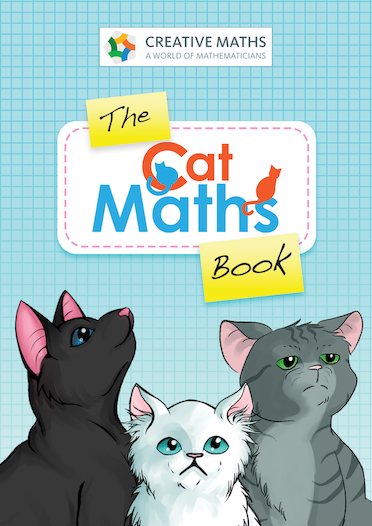Creative Maths
A world of mathematicians### Creative Maths

\$0.00 \$20.00

The Cat Maths Book provides a great introduction to using Cat Maths in the classroom or at home. This PDF version provides The Cat Maths Book as an ebook.

This product requires the use of The Cat Pack family game

The Cat Maths Book is a 36 page book which provides an introduction to the cat cards, activities, lessons and an outline of the skills developed. The Cat Maths Book includes:

• 12 introductory activities
• 8 small group activities
• 2 lesson plans
• 2 active games